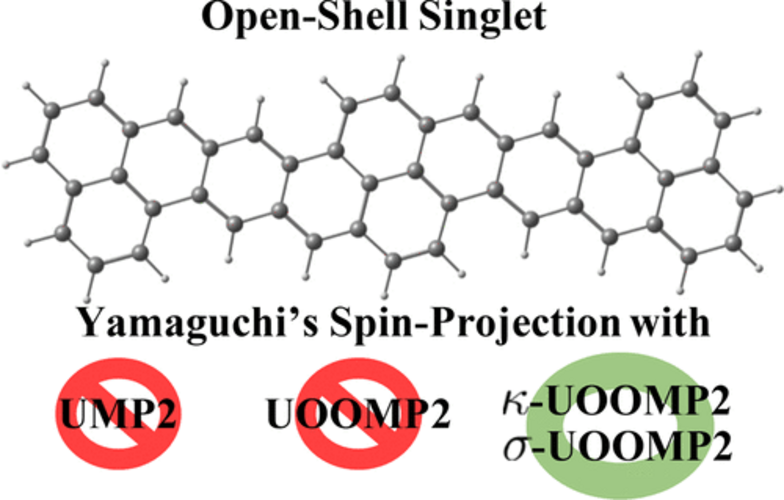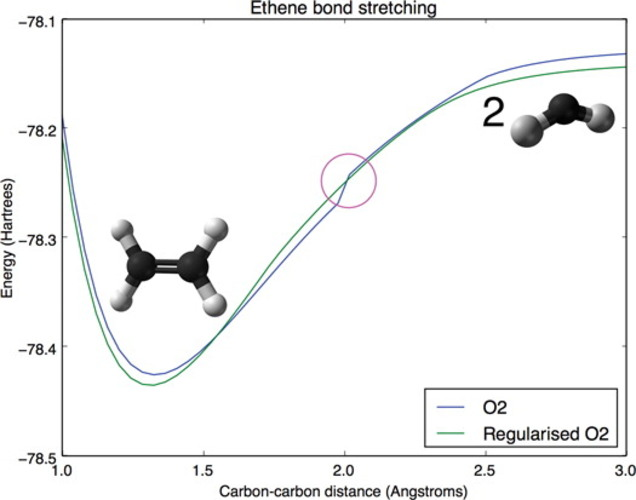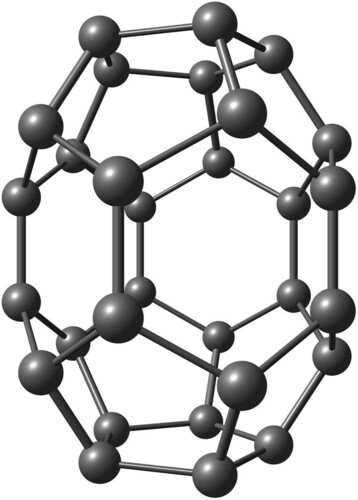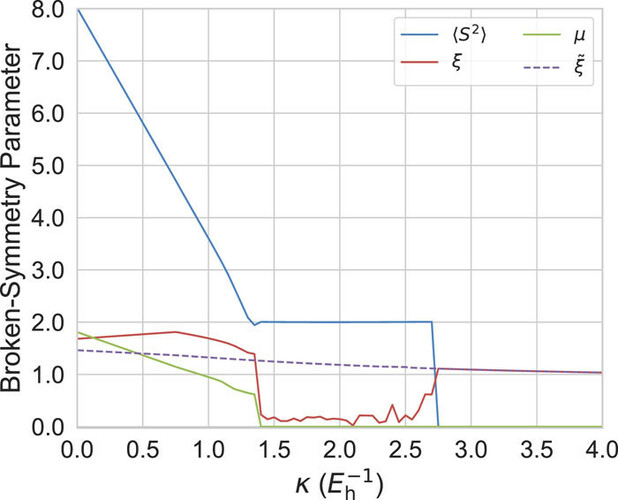# Regularized Orbital-Optimized Møller-Plesset Perturbation Theory for Strongly Correlated Species• Unrestricted Hartree-Fock (UHF) may exhibit artificial spin-symmetry-breaking in open-shell systems, and using UHF orbitals for correlated wave function calculations could lead to incorrect energetics and properties.

• Orbital-optimized MP2 (OOMP2) restores spin-symmetry and removes the discontinuity in the first-order properties at the onset of symmetry-breaking in MP2.

• Orbital optimization in OOMP2 could produce very small energy denominators which make the total energy diverge. In addition, OOMP2 may unphysically prefer restricted solutions and remove the Coulson-Fischer point from potential energy curves.

• Q-Chem offers several regularization schemes ($$\kappa$$-OOMP2 and $$\sigma$$-OOMP2) to fix the above issues. Combined with approximate spin-projection, these regularized OOMP2 methods are powerful and economical tools for studying strongly correlated systems.C$${30}$$ (D$${5h}$$ symmetry), a strongly correlated molecular cluster of which symmetry-breaking is essentialMeasures of symmetry breaking parameters as functions of the regularization strength of C$$_{30}$$